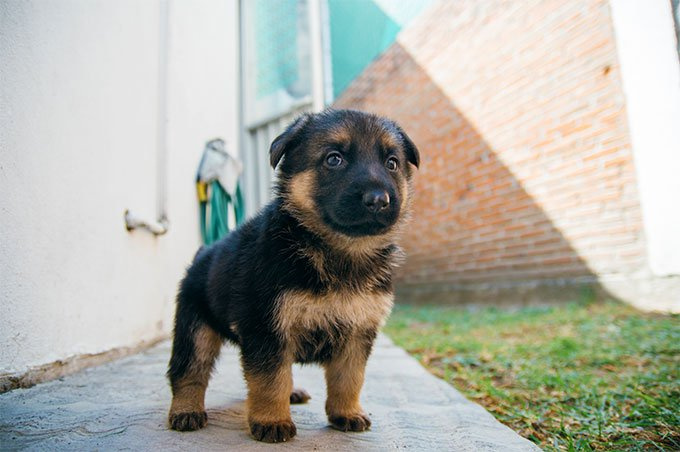# Prediction probabiliies for a bunch of test images

(Natalija Lace) #1

Hello everyone,

I am testing my trained models to see how accurate they are at predicting the categories of the brand new images (not seen previously). I used the following code, but there are 2 issues with it: a) it shows just one category without % probabilities; b) it is set up to just test one image at a time:

In: img = open_image(“Folder/xxx.png”)

pred_class, pred_idx, outputs = learn.predict(img);pred_class

Out: Category (Category name is given here)

My question is how can I test a bunch of brand new images and get the result with file names and percentage of probabilities for each of my 3 possible categories, i.e. the output should be as follows:

xxx.png - category A 98% category B 1.5% Category C 0.5%
ccc.png - category A 80% category B 10 % Category C 10%

something like that.

0 Likes

(Zachary Mueller) #2

Pred class will only have the class. If we follow the documentation for predictions here we can see that if we do:

``````img = data.train_ds
learn.predict(img)
``````

It will return: `(Category 3, tensor(0), tensor([0.5275, 0.4725]))`
So as we can see, in your case “outputs” will result in your raw probabilities. If you want multiple, generate a test dataset of your classes and add an `.add_test()` option for your dataset during databunch creation and run `learn.get_preds(DatasetType.Test)`

However also, if you add in a third class that was never trained on it will only try for two classes as you were never trained to search for three!1 Like

(Natalija Lace) #3

Thank you very much for the suggestion, do you mean I can add test dataset like this (or am I horribly wrong?):

path=Path(“My Folder”)
classes = [‘Class A’,‘Class B’,‘Class C’]
data = ImageDataBunch.single_from_classes(path, classes, .add.test(), size=(100,180)).normalize(imagenet_stats)
learn = create_cnn(data, models.resnet50)

pred_class, pred_idx, outputs = learn.get_preds(DatasetType.Test)`

I have 3 classes, and the model was trained on all 3, the test files also have one of these 3 classes assigned to them by observers, I just want to test how good is my model in terms of generalizing on something that belongs to one of these 3 categories, but has not been seen before.

Also, I need this for potential model deployment, so adding test files to train and valid files is not exactly what I need, I think.

0 Likes

(Zachary Mueller) #4

Got it!Here is the documentation for test sets, and there are examples in the course-v3 notebooks.

If you are doing for deployment, instead make an ImageList of your set of images you want to train on, and when you use `load_learner` for deployment, pass in that ImageList in.

1 Like

(Natalija Lace) #5

ok, so basically to get results on test set, I will have to add test set to my training and validation sets when I am training my model? Something like we did in v 1 when we used to have 3 sets of data in folders? (i.e. train, valid, test?) But in this new version I think we just have all data and tell the model how to separate it, i.e. valid_pct=0.3, for example?

Sorry, I am terribly confused about all this. I need to test a bunch of new files to see how accurate my model is and after that, I need to figure the way so my users could upload a bunch of files and get results back.

Some time ago someone did a dog classification on render (it is gone now https://whatdog.onrender.com/), where you upload the file and get results back, for example:Result = [(‘german_shepherd’, 0.29597368836402893), (‘border_terrier’, 0.0761934369802475), (‘rottweiler’, 0.04072378948330879)]

0 Likes

(Zachary Mueller) #6

If you have a test set you want to examine during training yes. During production (post learn.export()) no, you will not. You can pass in a test set during deployment and the call to `load_learner`.

We still are in valid_pct as well, we are saying split our dataset into 30% validation. I have an example notebook that goes over what you describe here as well. The main bit of code you want is the following:

``````data = ImageList.from_folder(path)
learn = load_learner(path, export_file_name, test=data)
y, _ = learn.get_preds(DatasetType.Test)
y = torch.argmax(y, dim=1)
preds = [learn.data.classes[int(x)] for x in y]
``````

A way to do this during training is when you make an image databunch you do `.add_test(ImageList.from_folder(folder))` (this is assuming your test data is in one folder)

1 Like

(Natalija Lace) #7

is this the name of my trained model? For my single file I now use the following:

learn = create_cnn(data, models.resnet50)

but in case of deployment it is replaced with ```
learn = load_learner(path, export_file_name, test=data)

Sorry If I am asking some dumb questions and thank you very much, I will now have to digest all this to see if I fully understand it,

0 Likes

(Natalija Lace) #8

ok, here is what I’ve got but something is still not right, as I get nothing in terms of predictions (I have 95 images in test folder):

path=Path(“MyPath”)
data = ImageList.from_folder(path)
learn = load_learner(path,‘export.pkl’, test=data)
predictions = learn.get_preds(ds_type=DatasetType.Test)

predictions= calculates something, but I get no results, nothing, empty space. I also tried the following:

y, _ = learn.get_preds(DatasetType.Test)
y = torch.argmax(y, dim=1)
preds = [learn.data.classes[int(x)] for x in y]

and it also returns nothing. I got no errors, just no results at all. What am I doing wrong here?

Another question is that the model was trained on normalized images, and when I predicted a single image, it was also normalized via:

data = ImageList.from_folder(path, classes, size=(100,180)).normalize(imagenet_stats)

but in this case when I get a bunch of images from the test folder, they are not normalized? Would it negatively affect the model performance on these not-normalized test images?

0 Likes